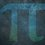# Fractional Proof Note $1$

Let's prove that:

$\frac{x}{100}$ $\times 100 = x$

$\frac{x}{100}$ $\times 100$

$\frac{100x}{100}$

$\frac{10x}{10}$

$\frac{1x}{1}$

$x$

Therefore, $\frac{x}{100}$ $\times 100 = x$Note by Yajat Shamji
9 months, 2 weeks ago

This discussion board is a place to discuss our Daily Challenges and the math and science related to those challenges. Explanations are more than just a solution — they should explain the steps and thinking strategies that you used to obtain the solution. Comments should further the discussion of math and science.

When posting on Brilliant:

• Use the emojis to react to an explanation, whether you're congratulating a job well done , or just really confused .
• Ask specific questions about the challenge or the steps in somebody's explanation. Well-posed questions can add a lot to the discussion, but posting "I don't understand!" doesn't help anyone.
• Try to contribute something new to the discussion, whether it is an extension, generalization or other idea related to the challenge.
• Stay on topic — we're all here to learn more about math and science, not to hear about your favorite get-rich-quick scheme or current world events.

MarkdownAppears as
*italics* or _italics_ italics
**bold** or __bold__ bold
- bulleted- list
• bulleted
• list
1. numbered2. list
1. numbered
2. list
Note: you must add a full line of space before and after lists for them to show up correctly
paragraph 1paragraph 2

paragraph 1

paragraph 2

[example link](https://brilliant.org)example link
> This is a quote
This is a quote
    # I indented these lines
# 4 spaces, and now they show
# up as a code block.

print "hello world"
# I indented these lines
# 4 spaces, and now they show
# up as a code block.

print "hello world"
MathAppears as
Remember to wrap math in $$ ... $$ or $ ... $ to ensure proper formatting.
2 \times 3 $2 \times 3$
2^{34} $2^{34}$
a_{i-1} $a_{i-1}$
\frac{2}{3} $\frac{2}{3}$
\sqrt{2} $\sqrt{2}$
\sum_{i=1}^3 $\sum_{i=1}^3$
\sin \theta $\sin \theta$
\boxed{123} $\boxed{123}$

## Comments

Sort by:

Top Newest

Maybe simple? Not quite...

$\frac{x}{100} × 100$

$= x × 100^{-1} × 100^1$

$= x × 100^{1-1}$

$= x × 100^0$

$= x × 1$

$= \boxed{x}$

- 9 months, 2 weeks ago

Log in to reply

it is not simpler

- 9 months, 2 weeks ago

Log in to reply

Yup, but I am just bored...

- 9 months, 2 weeks ago

Log in to reply

do my question (they are difficult)

- 9 months, 2 weeks ago

Log in to reply

- 9 months, 2 weeks ago

Log in to reply

More simpler :  $\frac{x}{100} \times 100 = x$

- 9 months, 2 weeks ago

Log in to reply

A simpler one: $\dfrac{x}{100}\times100=\dfrac{100x}{100}=\dfrac{100}{100}\times x=1\times x=x$

- 9 months, 2 weeks ago

Log in to reply

Nice!

- 9 months, 2 weeks ago

Log in to reply

×

Problem Loading...

Note Loading...

Set Loading...